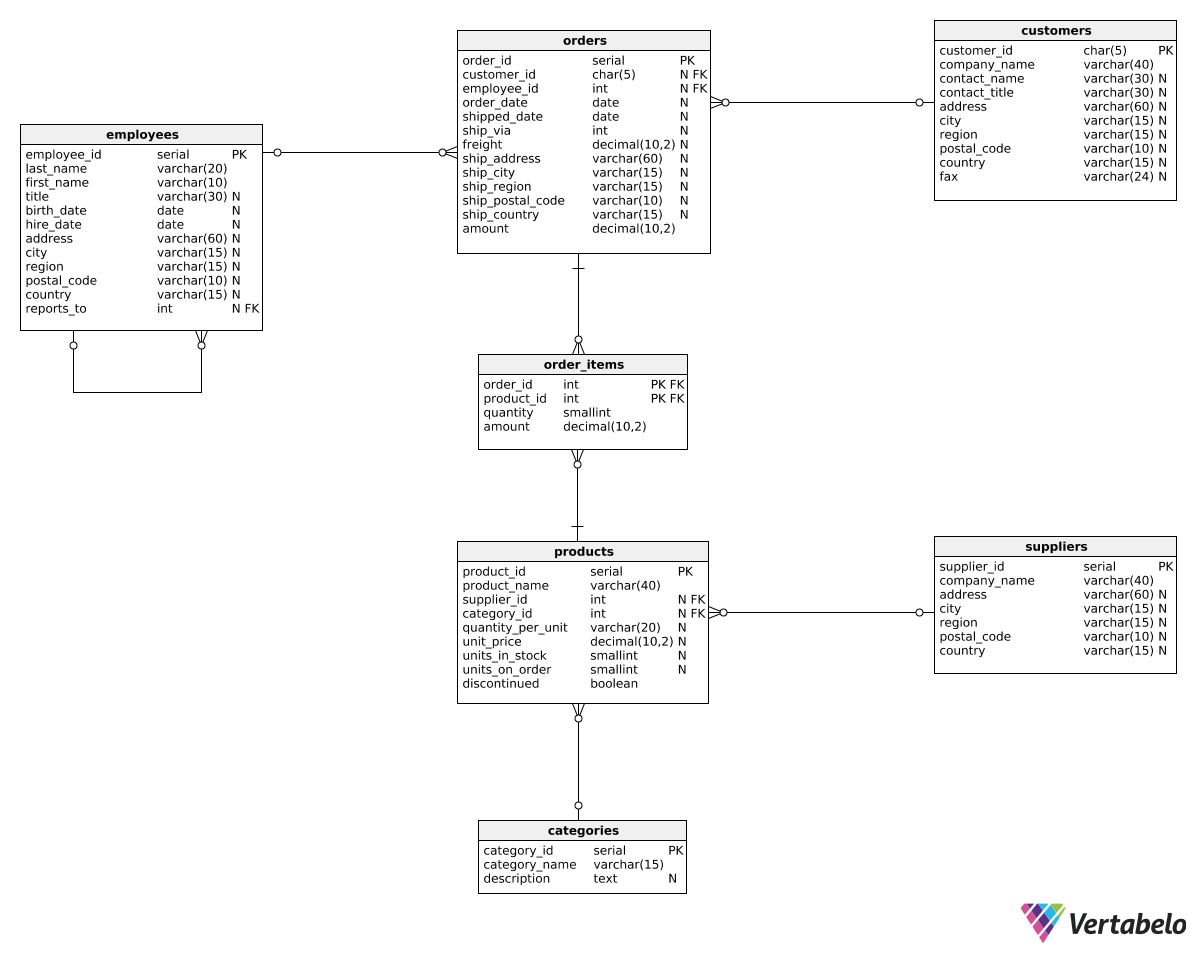Deals Of The Week - hours only!Up to 80% off on all courses and bundles.-Close
Introduction
Total revenue for multiple periods
Calculating deltas
Creating "revenue in quarters" reports
Summary

## Instruction

Awesome! We can also express the delta between two periods as a percentage. Look at the following query:

SELECT
EXTRACT(year FROM order_date) AS revenue_year,
SUM(amount) AS total_revenue,
ROUND(
100 *
(SUM(amount) - LAG(SUM(amount), 1)
OVER (ORDER BY EXTRACT(year FROM order_date)))
/ CAST(LAG(SUM(amount), 1) OVER (ORDER BY EXTRACT(year FROM order_date)) AS decimal(10, 2)),
2) AS delta_percentage
FROM orders
GROUP BY EXTRACT(year FROM order_date)
ORDER BY EXTRACT(year FROM order_date);


Here is the result:

revenue_year total_revenue delta_percentage
2016 218600.23 NULL
2017 655506.15 199.87
2018 273379.76 -58.29

This time, the calculation in the last column is a bit more complicated:

1. We divide the change between the current and previous period by the revenue value from the previous period.
2. To avoid integer division, we cast the denominator to a decimal type with:
CAST(LAG(SUM(amount), 1) OVER (ORDER BY EXTRACT(year FROM order_date)) AS decimal(10, 2)))
For larger numbers, you can of course increase the first argument of decimal(10,2).
3. We multiply the division result by 100 to get the percentage value.
4. We round the percentage to two decimal places using the ROUND(value, 2) function.

## Exercise

The template query contains the code from the previous example. Modify it to show the revenue change as a percentage that's rounded to three decimal places.

Here's how you can count the revenue change as a percentage:

\frac{\textrm{total amount} - \textrm{total amount from preceding quarters}}{\textrm{total amount from preceding quarters}} \cdot 100

Remember to round the result to three decimal places.

### Stuck? Here's a hint!

Use the following expression to calculate the last column:

ROUND(
100 *
(SUM(amount) - LAG(SUM(amount), 1)
OVER (ORDER BY EXTRACT(year FROM order_date),
EXTRACT(quarter FROM order_date)))
/ CAST(LAG(SUM(amount), 1)
OVER (ORDER BY EXTRACT(year FROM order_date),
EXTRACT(quarter FROM order_date)) AS decimal(10, 2)),
3) AS delta